Slides

# How I see our roles

Professors: Guide students on what is impossible, possible, and hints on what might be possible

Ph.D students: Venture into the unknown and make something (more) possible

Master students: Learn all that is currently possible

Bachelor students: Learn how to make complicated into easy

Industry: Take what is possible, and/or complicated, and make it easyThe world is analog. The laws of behavior are written in the mathematics of calculus 1

$$\oint_{\partial \Omega} \mathbf{E} \cdot d\mathbf{S} = \frac{1}{\epsilon_0} \iiint_{V} \mathbf{\rho} \cdot dV$$
Relates net electric flux to net enclosed electric charge

$$\oint_{\partial \Omega} \mathbf{B} \cdot d\mathbf{S} = 0$$ Relates net magnetic flux to net enclosed magnetic charge

$$\oint_{\partial \Sigma} \mathbf{E} \cdot d\mathbf{\ell} = - \frac{d}{dt}\iint_\Sigma \mathbf{B} \cdot d\mathbf{S}$$ Relates induced electric field to changing magnetic flux

$$\oint_{\partial \Sigma} \mathbf{B} \cdot d\mathbf{\ell} = \mu_0\left( \iint_\Sigma \mathbf{J} \cdot d\mathbf{S} + \epsilon_0 \frac{d}{dt}\iint_\Sigma \mathbf{E} \cdot d\mathbf{S} \right)$$ Relates induced magnetic field to changing electric flux and to current density

The behavior of electrons is written in quantum mechanics

$$\psi(x,t) = Ae^{j(kx - \omega t)}$$ Probability amplitude of an electron

$$\frac{1}{2 m} \frac{\hbar}{j^2} \frac{\partial^2}{\partial^2 x}\psi(x,t) + U(x)\psi(x,t) = -\frac{\hbar}{j}\frac{\partial}{\partial t} \psi(x,t)$$ Space and time evolution of an electron (Schrödinger equation)

$$n = \int_{E_1}^{E_2}{N(E)f(E)dE}$$ Electron density (Density of states)

$$f(E) = \frac{1}{e^{(E_i - E_F)/kT} + 1}$$ Relates the average number of fermions in thermal equlilibrium to the energy of a single-particle state (Fermi-Dirac statistics)

[.table-separator: #000000, stroke-width(1)] [.table: margin(8)]

The abstract digital world is written in the mathematics of boolean algebra2

$$1 = \text{True}$$, $$0 = \text{False}$$

A B NOT(A AND B)
0 0 1
0 1 1
1 0 1
1 1 0

All digital processing can be made with the NOT(A AND B) function!

# People that design digital circuits can reuse the work of others# People that design analog circuits can learn from others, but need to deal with the real world on their own### Should we do as much as possible in the abstract digital world?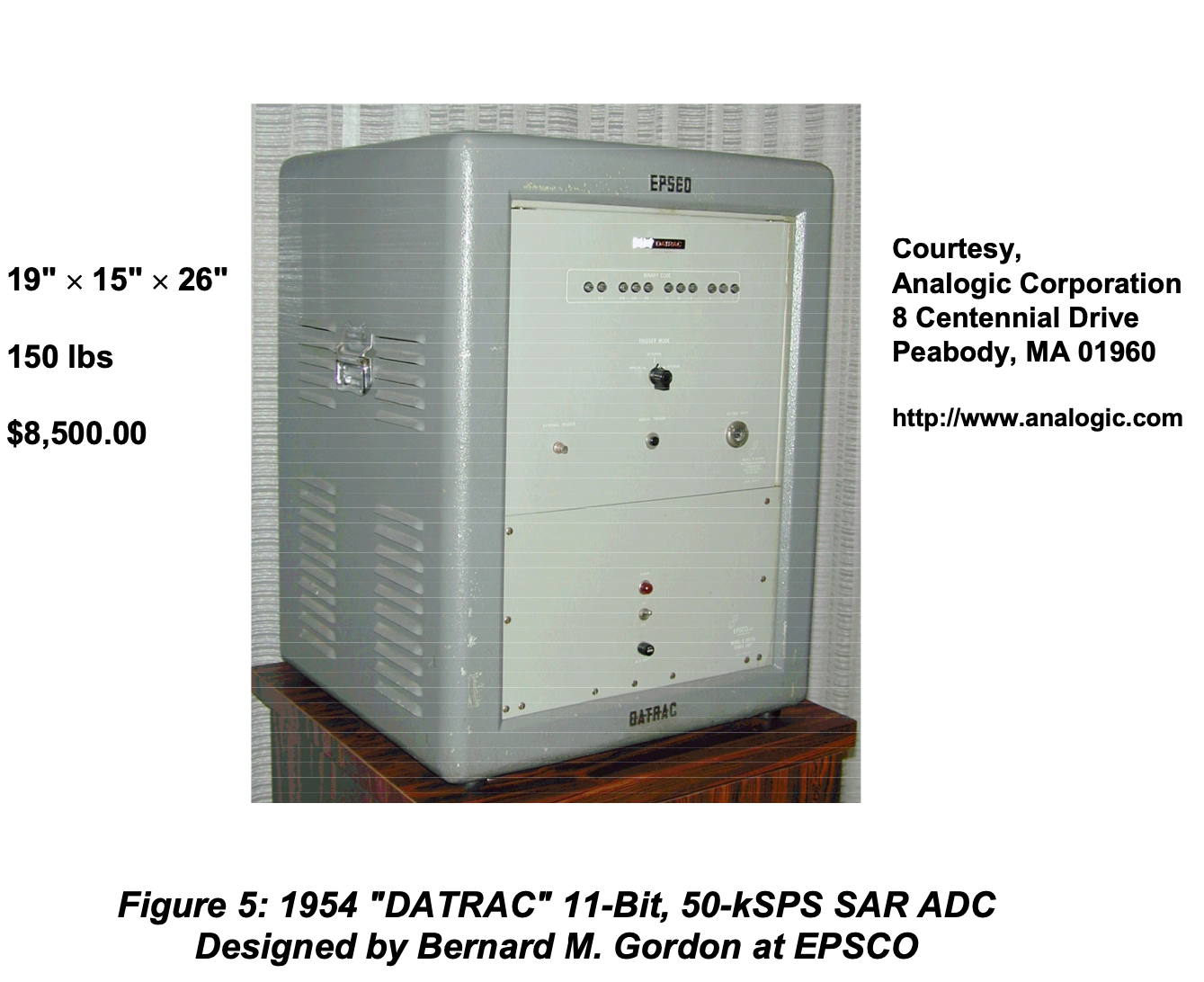One of the first commerical offerings of a successive approximation analog-to-digital converter 3

# Why is reuse of analog circuits hard?

### Life of an analog designer: Schematic Design### Life of an analog designer: Layout Design# My journey on “How can I simplify analog design?”

## Trigger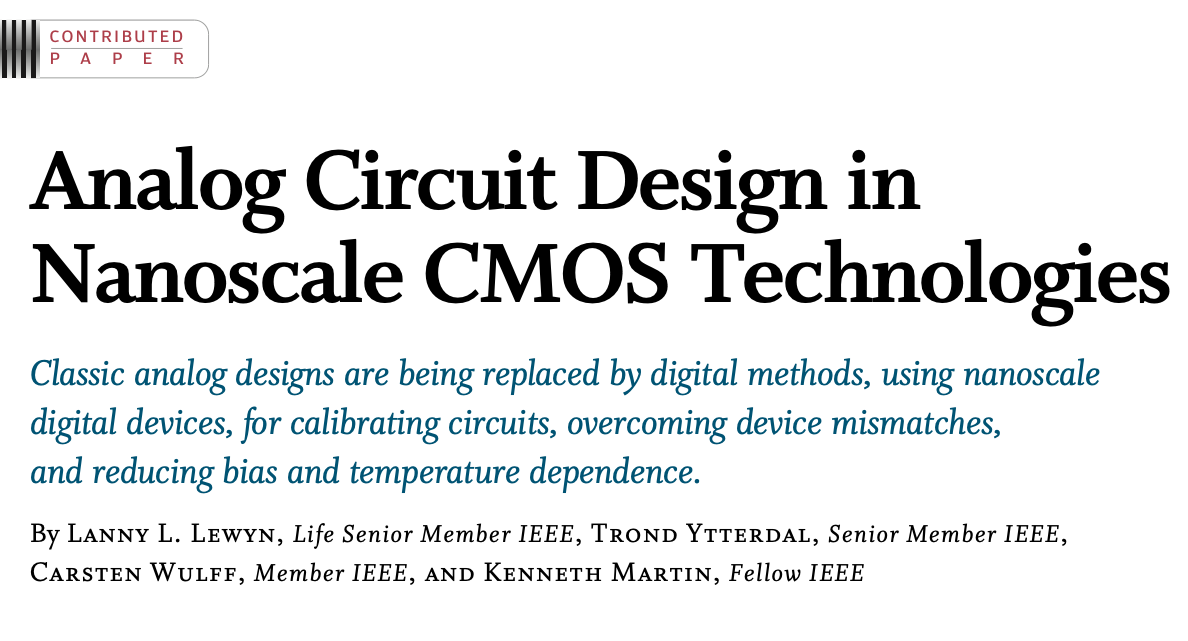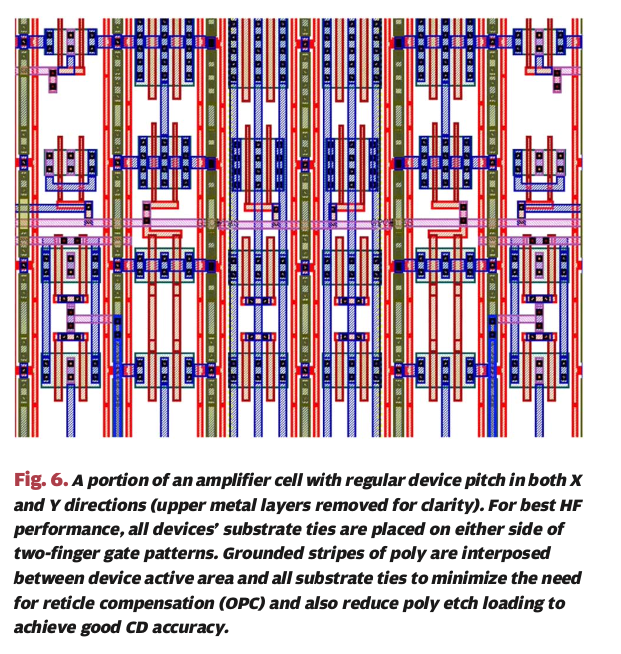## Problem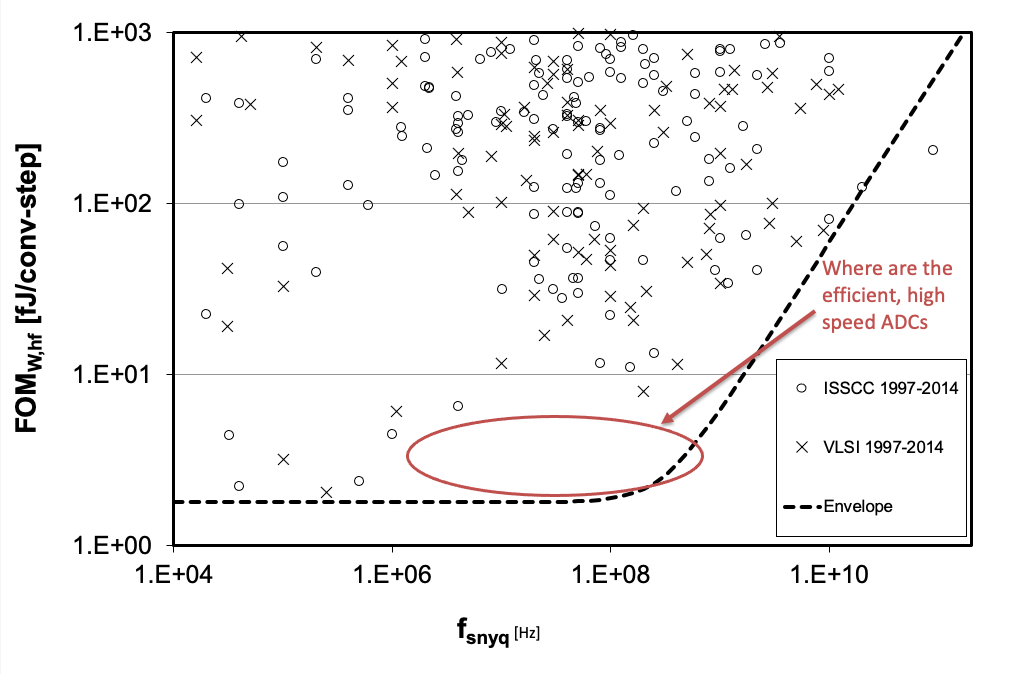## Architecture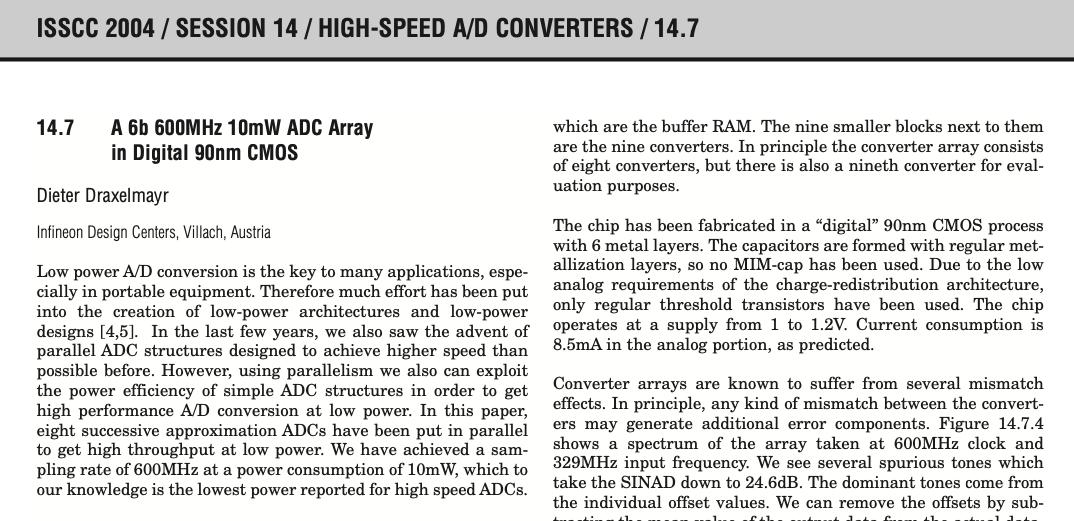## Plan

9-bit SAR ADC with 28 nm FDSOI transistors

9-bit SAR ADC with IO voltage (180 nm) FDSOI transistors

# How to make multiple SAR ADCs with limited time?

Spend 50% of time for 6 months to develop a tool to make SAR ADCs

Spend 50% of time for 6 months to make the SAR ADCs16 k Perl lines. Ported to C++ for speed $$\Rightarrow$$ ciccreatorA Compiled 9-bit 20-MS/s 3.5-fJ/conv.step SAR ADC in 28-nm FDSOI for Bluetooth Low Energy Receivers# Since then

Measured: 28 nm FDSOI, 55 nm Ported: 22 nm FDSOI, 22 nm, 28 nm, 65 nm, 130 nm

Finally, there is an open source port to skywater 130nm! wulffern/sun_sar9b_sky130nm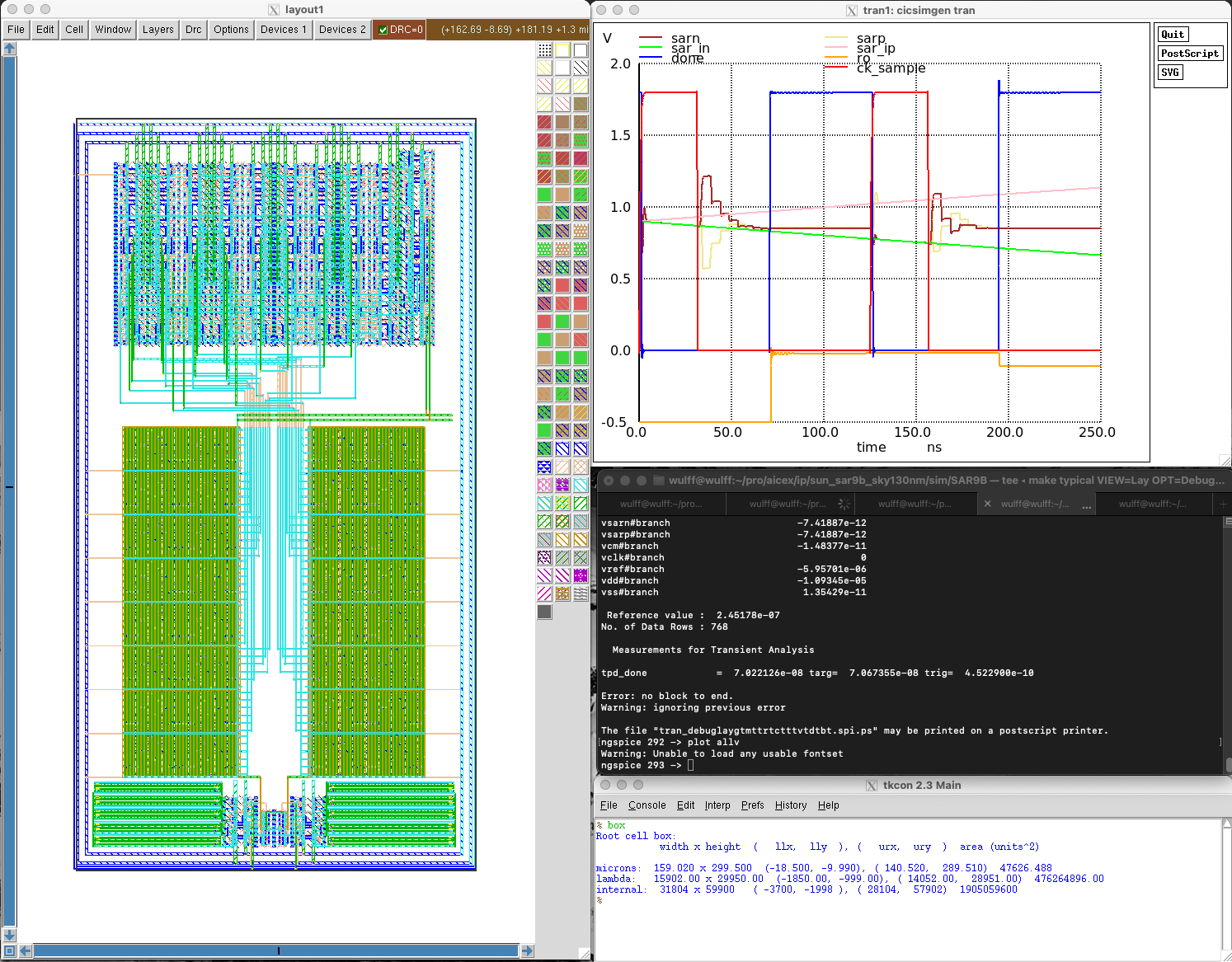# Super simple transistor was a good choice for portability

        { "name" : "DMOS_BULKN" ,
"class" : "Gds::GdsPatternTransistor",
"abstract" : 1,
"yoffset": -0.5,
"widthoffset" : -0.5,
"fillCoordinatesFromStrings" : [
[   "OD",
"-------------------",
"----xxx------------",
"----xxx------------",
"----xxx------------",
"-------------------"
],
...
[   "M1",
"----------------xxx",
"----wDw---------xxx",
"----------wGw---xBx",
"----wSw---------xxx",
"----------------xxx"
],
...
[   "NDIFFC",
"-------------------",
"----LTR------------",
"-------------------",
"----LTR------------",
"-------------------"
]
]
}

{ "name" : "DMOS" ,
"class" : "Gds::GdsPatternTransistor",
"yoffset": -0.5,
"type": "pch",
"widthoffset" : -1,
"fillCoordinatesFromStrings" : [
[  "OD",
"------------------xxxx",
"----xxK-----------xCxC",
"----xxx-----------xxxx",
"----xxK-----------xCxC",
"------------------xxxx"
],
[  "PO",
"-mmmmmmmmmmmmm--------",
"----------------------",
"-mmmmmmmmmmcxc--------",
"----------------------",
"-mmmmmmmmmmmmm--------"
],
[  "M1",
"------------------xxxx",
"----wDww----------xxxx",
"-----------wGww---xBxx",
"----wSww----------xxxx",
"------------------xxxx"
]
],
"afterNew" : {
"copyColumns" :[
{ "count" : 0, "offset" : 4,"length" : 4}
]
}
}


2016 (Perl compiler)

{ "name": "SARCMPHX1_CV",
"description" : "Half a strong-arm comparator",
"class" : "Layout::LayoutDigitalCell",
"setYoffsetHalf" :  "" ,
"rows" : 7,
"beforeRoute" : {
["M1","VMR","MP4:G||MP6:G"],
["M1","CI","MN1:G||MN5:G"],
["M1","N2","MN1:D,MN3:D,MN5:D-|--MP1:D"],
["M1","N1","MN0:D,MN2:D|-MN4:D"],
["M1","N1","MN0:D-|--MP0:S"],
["M1","CO","MP3:D,MP5:D--|-MN6:D"],
["PO","CK","MN0:G-MP0:G"],
["M1","CK","MP0:G,MP1:G-|MP3:G"],
["M4","NC","MP2$:D--|--MP2:G"] ] }, "afterRoute" : { "addPortOnRects" : [ ["AVDD","M4" ], ["N1","M1","MN4:D"], ["N2","M1","MN5:D" ]] } }  2022 (C++ compiler) { "name": "SARCMPHX1_CV", "description" : "Half a strong-arm comparator", "class" : "Layout::LayoutDigitalCell", "setYoffsetHalf" : 1 , "rows" : 7, "meta" : { "noSchematic" : true }, "decorator" : [ {"ConnectSourceDrain" : ["M1","||",""]} ], "beforeRoute" : { "addDirectedRoutes" : [ ["PO","VMR","MN6:G-MP6:G"], ["M1","VMR","MP4:G||MP6:G"], ["M1","CI","MN1:G||MN5:G"], ["M1","N2","MN1:D,MN3:D,MN5:D-|--MP1:D"], ["M1","N1","MN0:D,MN2:D|-MN4:D"], ["M1","N1","MN0:D-|--MP0:S"], ["M1","CO","MP3:D,MP5:D--|-MN6:D"], ["PO","CK","MN0:G-MP0:G"], ["M1","CK","MP0:G,MP1:G-|MP3:G"], ["M4","NC","MP2$:D-|--MP2:G"]
]
},
"afterRoute" : {
["BULKN","M1"],
["AVDD","M4" ],
["N1","M1","MN4:D"],
["N2","M1","MN5:D" ]]
}
}



# Usage is hard, requires a new type of analog designer/programmer

## wulffern/aicex

1. https://www.analog.com/media/en/training-seminars/tutorials/mt-021.pdf